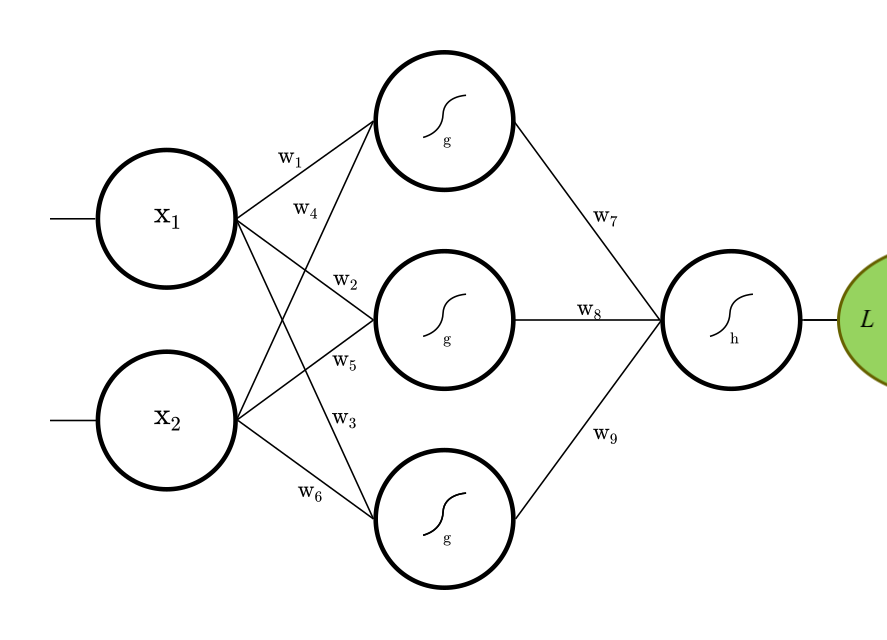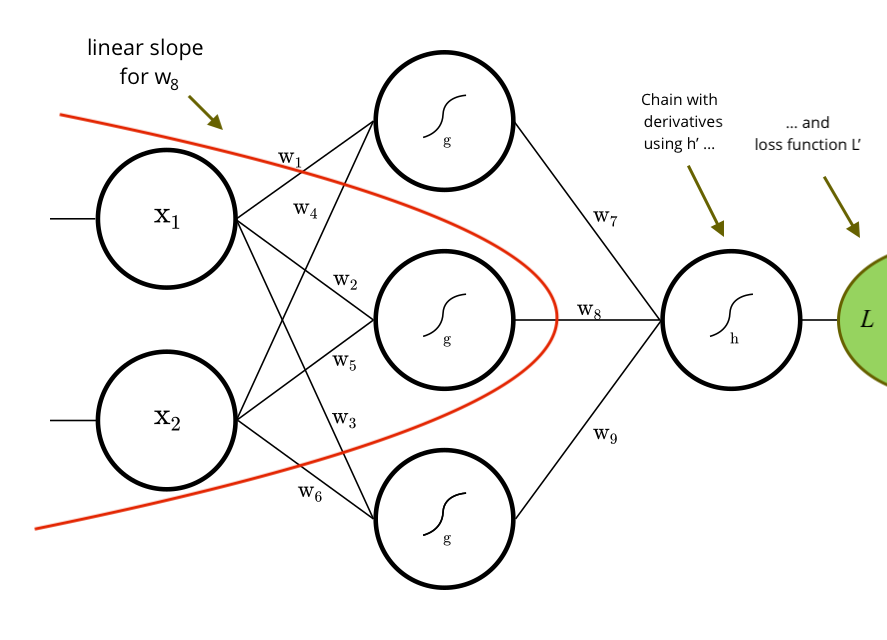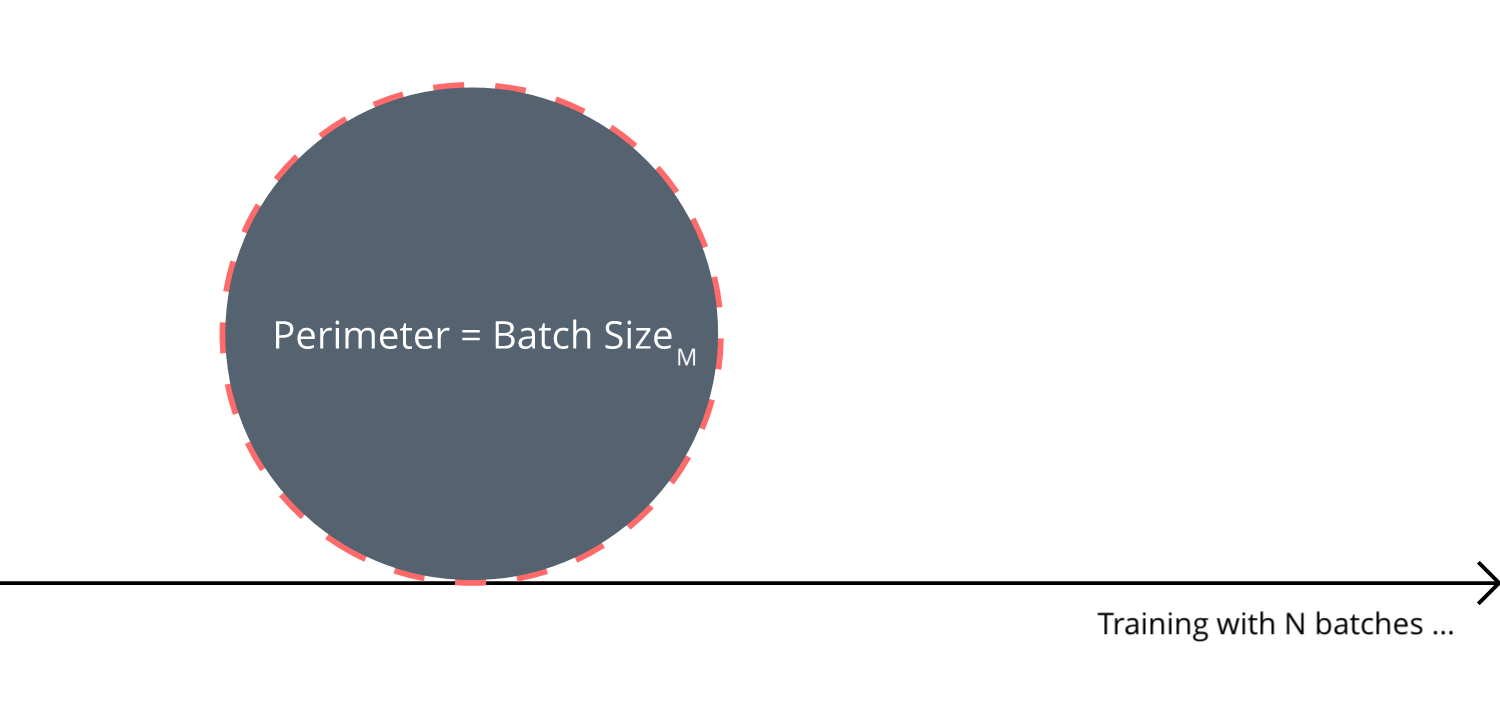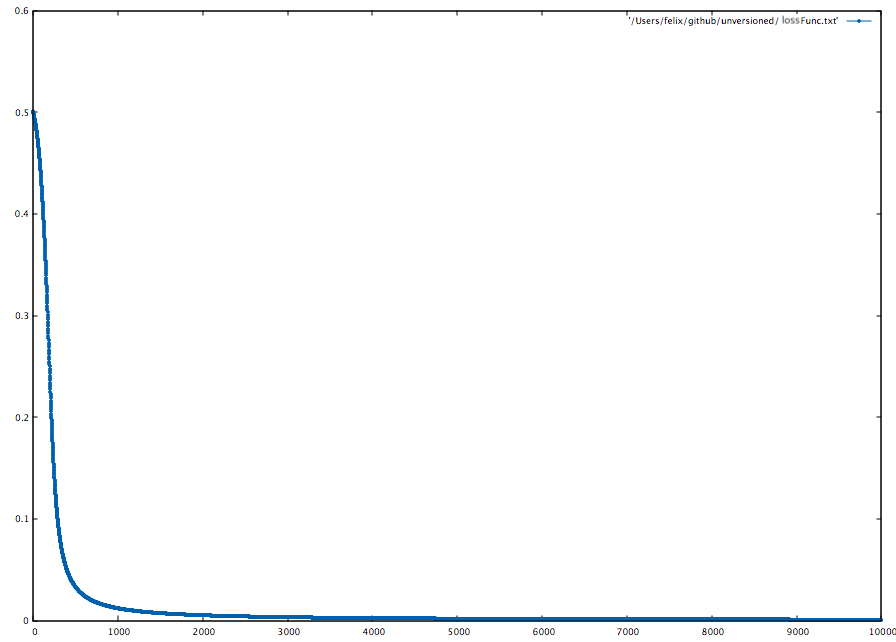# zenecture / neuroflow

Artificial Neural Networks for ScalaNeuroFlow is a library to design, train and evaluate Artificial Neural Networks.

# Getting Started

The library aims at ease of use, keeping things intuitive and simple. Neural Nets bring joy into your project, a journey full of experiments. :o)

There are three modules:

• core: building blocks for neural networks
• application: plugins, helpers, functionality related to application
• playground: examples how to use it

To use NeuroFlow for Scala 2.12.x, add these dependencies to your SBT project:

```libraryDependencies ++= Seq(
"com.zenecture"   %%   "neuroflow-core"          %   "1.7.8",
"com.zenecture"   %%   "neuroflow-application"   %   "1.7.8"
)

resolvers ++= Seq(
"neuroflow-libs" at "https://github.com/zenecture/neuroflow-libs/raw/master/"
)```

If you are new to the math behind Neural Nets, you can read about the core principles here:

Seeing code examples is a good way to get started. You may have a look at the playground for basic inspiration.

# Construction of a NetLet's construct the fully connected feed-forward net (FFN) depicted above.

```import neuroflow.core.Activators.Double._
import neuroflow.core._
import neuroflow.dsl._
import neuroflow.nets.cpu.DenseNetwork._

implicit val weights = WeightBreeder[Double].normal(μ = 0.0, σ = 0.1)

val (g, h) = (Sigmoid, Sigmoid)

val net = Network(
layout = Vector(2) :: Dense(3, g) :: Dense(1, h) :: SquaredError()
)```

This gives a fully connected `DenseNetwork` under the `SquaredError` loss function, running on CPU. The `weights` are drawn from normal distribution by `WeightBreeder`. We have predefined activators and place a softly firing `Sigmoid` on the cells.

A full model is expressed as a linear `Layout` graph and a `Settings` instance. The layout is implemented as a heterogenous list, allowing compile-time checks for valid compositions. For instance, a little deeper net, with some rates and rules defined, could look like this:

```val (e, f) = (Some(Linear.biased(0.1)), ReLU)

val L =
Vector   (11, e)      ::
Dense    ( 3, f)      ::
Dense   (180, f)      ::
Dense   (360, f)      ::
Dense   (420, f)      ::
Dense   (314, f)      ::
Dense   (271, f)      ::
Dense    (23, f)      ::    SoftmaxLogEntropy()

val deeperNet = Network(
layout = L,
settings = Settings[Double](
updateRule = Vanilla(),
batchSize = Some(8),
iterations = 256,
learningRate = {
case (iter, α) if iter < 128 => 1E-4
case (_, _)  => 1E-6
},
precision = 1E-8
)
)```

Here, the `SoftmaxLogEntropy` layer computes loss and gradient, which is backpropped into the last `Dense` layer of the net. The `updateRule` defines how weights are updated for gradient descent. The `batchSize` defines how many samples are presented per weight update. The `learningRate` is a partial function from current iteration and learning rate producing a new learning rate. Training terminates after `iterations`, or if loss satisfies `precision`.

Another important aspect of the net is its numerical type. For example, on the GPU, you might want to work with `Float` instead of `Double`. The numerical type is set by explicitly annotating it on both the `WeightBreeder` and `Settings` instances.

Have a look at the `Settings` class for the complete list of options.

# Training

Our small `net` is a function `f: X -> Y`. It maps from 2d-vector `X` to 1d-vector `Y`. There are many functions of this kind to learn out there. Here, we go with the XOR function. It is linearly not separable, so we can check whether the net can capture this non-linearity.

To learn, we need to know what it means to be wrong. The `SquaredError` loss function is defined as follows:

``````SquaredError(X, Y, W) = Σ1/2(Y - net(X, W))²
``````

Where `W` are the weights, `Y` is the target and `net(X, W)` the prediction. The sum `Σ` is taken over all samples and the square `²` gives a convex functional form. We interpret the XOR-adder as a regression challenge, so the `SquaredError` is our choice. Alternatively, for 1-of-K classification, we could use the `SoftmaxLogEntropy` loss function, for N-of-K `SoftmaxLogMultEntropy` respectively.Example: Derivative for w8

In NeuroFlow, we work with Breeze, in particular with `DenseVector[V]` and `DenseMatrix[V]`. Let's define the XOR training data using in-line vector notation:

```import neuroflow.application.plugin.Notation._

val xs = Seq(->(0.0, 0.0), ->(0.0, 1.0), ->(1.0, 0.0), ->(1.0, 1.0))
val ys = Seq(->(0.0), ->(1.0), ->(1.0), ->(0.0))

/*
It's the XOR-Function :-).
Or: the net learns to add binary digits modulo 2.
*/

net.train(xs, ys)```

And then we call `train` on `net` to start.

# Monitoring

The training progress is printed on console so we can track it.

```[run-main-0] INFO neuroflow.nets.cpu.DenseNetworkDouble - [14.01.2018 22:26:56:188] Training with 4 samples, batch size = 4, batches = 1 ...
[run-main-0] INFO neuroflow.nets.cpu.DenseNetworkDouble - [14.01.2018 22:26:56:351] Iteration 1.1, Avg. Loss = 0,525310, Vector: 0.5253104527125074
[run-main-0] INFO neuroflow.nets.cpu.DenseNetworkDouble - [14.01.2018 22:26:56:387] Iteration 2.1, Avg. Loss = 0,525220, Vector: 0.5252200280272876
...```

One line is printed per iteration, `Iteration a.b` where `a` is the iteration count, `b` is the batch and `Avg. Loss` is the mean of the summed batch loss `Vector`. The batch count `b` loops, depending on the batch size, whereas the iteration count `a` progresses linearly until training is finished.Rolling over all samples with mini-batches (M:N).

To visualize the loss function, we can iteratively append the `Avg. Loss` to a `file` with `LossFuncOutput`.

```Settings(
lossFuncOutput = Some(LossFuncOutput(file = Some("~/NF/lossFunc.txt")))
)```

Now we can use beloved gnuplot:

```gnuplot> set style line 1 lc rgb '#0060ad' lt 1 lw 1 pt 7 ps 0.5
gnuplot> plot '~/NF/lossFunc.txt' with linespoints ls 1```Another way to visualize the training progress is to use the `LossFuncOutput` with a function `action: Double => Unit`.

```Settings(
lossFuncOutput = Some(LossFuncOutput(action = Some(loss => sendToDashboard(loss))))
)```

This function gets executed in the background after each iteration, using the `Avg. Loss` as input. One example is sending the loss to a real-time TV dashboard.

### Useful JVM args

```-Dorg.slf4j.simpleLogger.defaultLogLevel=debug # for misc runtime sys infos and gpu memory
-Xmx24G # example to increase heap size```

# Evaluation

When training is done, the net can be evaluated like a regular function:

```val x = ->(0.0, 1.0)
val result = net(x)
println(result) // DenseVector(0.9940081702899719)```

The resulting vector has dimension = 1, as specified for the XOR-example.

### Focusing

We can put focus on a layer and use it as the actual model output, instead of the last layer. For instance, here is a simple AutoEncoder `ae`:

```import neuroflow.dsl.Implicits._

val b = Dense(5, Linear)
val L = Vector(23) :: b :: Dense(23, Sigmoid) :: AbsCubicError()
val ae = Network(layout = L)

ae.train(xs, xs)```

It learns the input identity, but we are interested in the 5-dimensional activation from bottleneck layer `b` to produce a simple, compressed version of the input.

```val focused = ae focus b
val result = focused(->(0.1, 0.2, ..., 0.23))
println(result) // DenseVector(0.2, 0.7, 0.1, 0.8, 0.2)```

The `focus` on layer `b` gives a function, which can be applied just like the net it stems from. The type signature of the function is derived from the focused layer's algebraic type.

Another scenario where focusing is useful is when weights are initialized, i. e. the activations of the layers can be watched and adjusted to find good values, if a JVM debugger can't be attached.

# Extending

### Activators

A neural net consists of matrix multiplications and function applications. Since matrix multiplication is inherently linear here, all non-linearity has to come from the cells activators. The predefined ones are common and should be sufficient for most data, but at times special functions are required. Here is an example how to define your own:

```val c = new Activator[Double] {
val symbol = "My linear activator"
def apply(x: Double): Double = x + 0.1
def derivative(x: Double): Double = 1.0
}```

Then just drop it into a layer, e. g. `Dense(3, c)`. Luckily, the CPU implementation is flexible to run arbitrary code. If you need custom activators for GPU, you need to fork NF and implement them in CUDA.

### Loss Functions

You can tackle a lot of challenges by using the predefined loss functions. However, as with the activators, at times you need your own loss function, `Y, X -> Loss, Gradient`, so here is how to write one, for both CPU and GPU:

```val myLoss = new LossFunction[Double] {
def apply(y: DenseMatrix[Double], x: DenseMatrix[Double])(implicit /* operators ... */): (DenseMatrix[Double], DenseMatrix[Double]) = (loss, gradient) // CPU
def apply(y: CuMatrix[Double], x: CuMatrix[Double])(implicit /* operators ... */): (CuMatrix[Double], CuMatrix[Double]) = (loss, gradient) // GPU
}```

The targets `y` and predictions `x` are given input to produce `loss` and `gradient`, which will be backpropagated into the raw output layer. The batch layout is row-wise, so you need to work with the matrices accordingly. Don't fear the long implicit parameters when implementing the trait, these operators come from Breeze and should be just fine. Also look at the predefined loss functions for a starting point how to work with them.

`implicit def evidence[P <: Layer, V]: (P :: myLoss.type) EndsWith P = new ((P :: myLoss.type) EndsWith P) { }`

To use your loss function with the front-end DSL, you need to provide `evidence` for compile time checks.

```val L = Vector(3) :: Dense(3, Tanh) :: Dense(3, Tanh) :: myLoss
val net = Network(layout = L, settings)```

Alternatively, you can work with the corresponding net implementation, passing the layout directly without any checks.

# Using GPU

If your graphics card supports nVidia's CUDA (Compute Capability >= 3.0), you can train nets on the GPU, which is recommended for large nets with millions of weights and samples. On the contrary, smaller nets are faster to train on CPU, because while NeuroFlow is busy copying batches between host and GPU, CPU is already done.

To enable the GPU, you have to install the CUDA driver and toolkit (0.8.x). Example for Linux (Ubuntu 16.04):

```curl -O http://developer.download.nvidia.com/compute/cuda/repos/ubuntu1604/x86_64/cuda-repo-ubuntu1604_8.0.61-1_amd64.deb
sudo dpkg -i ./cuda-repo-ubuntu1604_8.0.61-1_amd64.deb
sudo apt-get update
sudo apt-get install cuda
sudo apt-get install cuda-toolkit-8-0```

With both driver and toolkit installed, you can import a GPU implementation for your model:

`import neuroflow.nets.gpu.DenseNetwork._`

# Persistence

We can save and load weights from nets with `neuroflow.application.plugin.IO`.

```import neuroflow.application.plugin.IO._

val file = "/path/to/net.nf"
implicit val weights = File.weightBreeder[Double](file)
val net = Network(layout, settings)
File.writeWeights(net.weights, file)
val json = Json.writeWeights(net.weights)```

The implicit `weights` to construct `net` come from `File.weightBreeder`. To save the weights back to `file`, we use `File.writeWeights`. The weight matrices are encoded in binary format.

To write into a database, we can use `Json.writeWeights` to retrieve a raw JSON string and fire a SQL query with it.

### Waypoints

```Settings(
waypoint = Some(Waypoint(nth = 3, (iter, weights) => File.writeWeights(weights, s"weights-iter-\$iter.nf")))
)```

It is good practice to use the `Waypoint[V]` option for nets with long training times. The training process can be seen as an infinitely running wheel, and with waypoints we can harvest weights now and then to compute intermediate results. Another reason to use it is when something crashes, saved weights allow continuation of training from a recent point. Here, every `nth = 3` step, the waypoint function is executed, receiving as input iteration count and a snapshot of the weights, which is written to file using `File.writeWeights`.